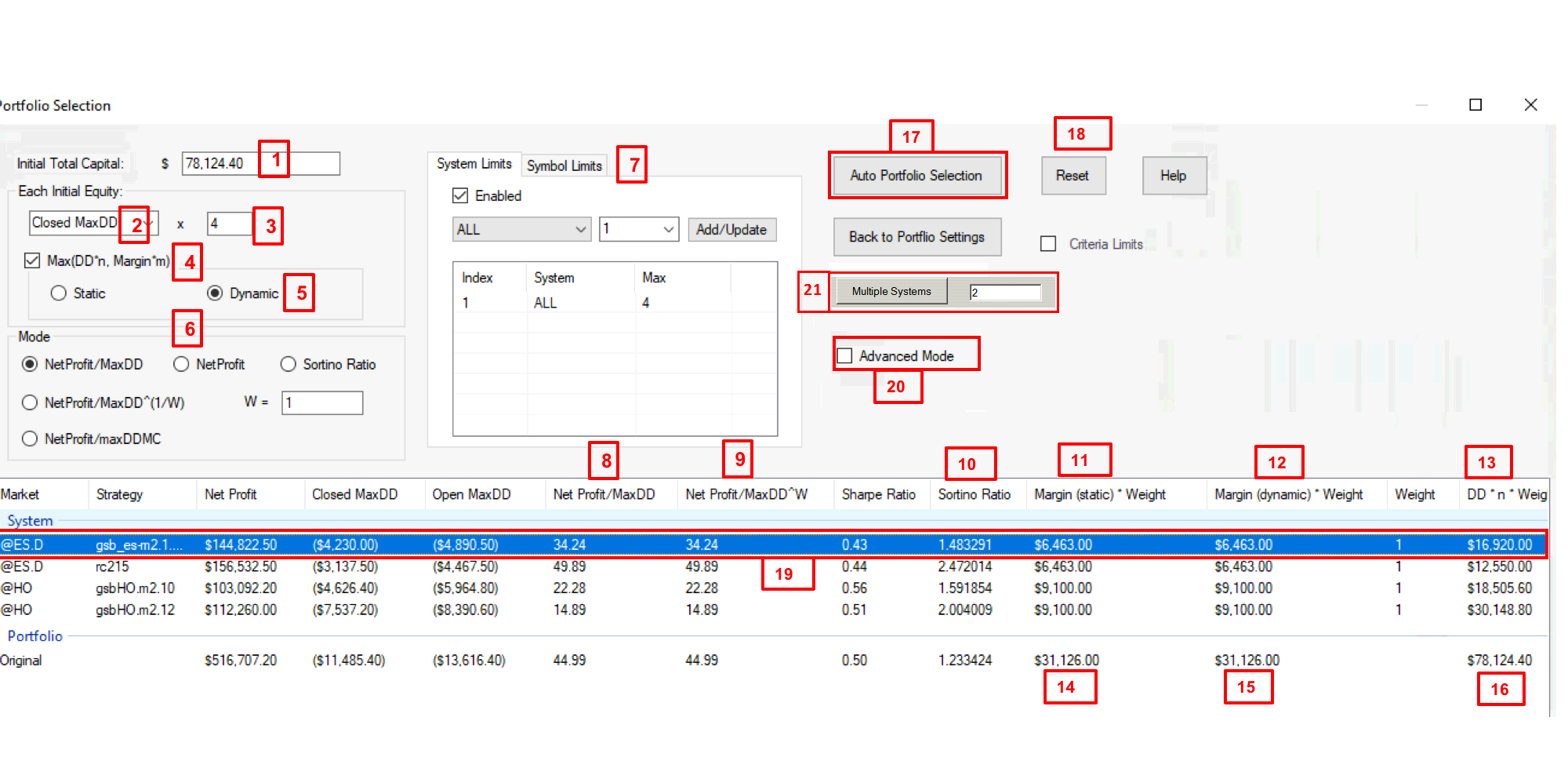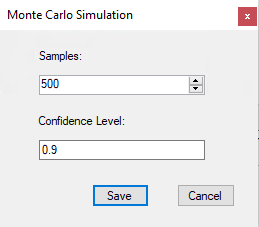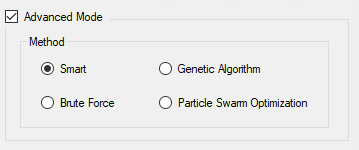Portfolio SelectionWhen you select a portfolio and generate a report you can Switch to “Portfolio Selection Page”

Note this is a different portfolio to above. Systems that trade infrequently were chosen to highlight the difference between dynamic and static margin.

1) The margin PA thinks this portfolio needs is \$78124. This is derived from field 16.

2) We can use close Max draw down of all systems, or the open trade or the average of the two figures. For overnight systems I recommend use open trade draw down.

3) 4 is the default. If you don't want to sue this feature set it to 0.1

4) As you can't trade more than what margin your broker gives you, the portfolio will not exceed margin requirements, and draw down * 4. If you don't want to use draw down for capital allocation set it to 1.

5) Static margin assumes you will have margin should every system trade at the same time.

Dynamic margin assumes that not all systems trade at the same time, so we can find the max margin figure that was used in past performance. Note one of the many dangers in exceed this is that on big moves, many systems trade & you get high chance of winning trades. On small moves the chance of loosing is much higher and few systems trade. This means trading > margin leads you getting all loosing trades and missing out on some big winning trades.

6) Net profit /Max draw down -  is the default metric. It seeks to build a portfolio that maximizes this ratio.

Net profit /Max draw down^(1/W) – If “W” is increase, the portfolio will give higher net profit at the expenses of Drawdown. W being 2 is a good defauly value.

Net profit /Max draw down Monte Carlo – If you chose this, you would have to select Money Carlo Simulation & Confidence Level.Net profit – This well maximize net profit. This will put all your portfolio in the most profitable system unless you add symbol limits and use the Max weight features on the Entry page of PA. This is not good as there is no diversification and your risk is high.

To fix this you can use symbol limits (see 7 below) and or use the max weight feature on the first page to not allow more than x contracts on any one system.

Sortino ratio. This seeks to maximize the Sortino ratio. Sortino is basically the sharp ratio of losing trades. This is an experimental concept.

7) This will limit the amount of contracts on any or all systems. You can choose any symbols you like, or set a global maximum with all. ie. If EF is set 3, there can never be

8) This is the net profit / draw down ratio.

9) The Net profit / SQR(draw down)/ This will place more emphasis on net profit at the expense of draw down. You may need to use symbol limits or limit the max number of contracts in the first screen of PA.

10) Sortino ratio of each system and the portfolio. High number is best as with other metrics.

11) Total static margin for each system and the entire portfolio. If margin for one system is \$6,000 and weight is 2, this figure will be \$12,000

12) Dynamic margin assumes that not all systems trade at the same time. We can use less margin as not all systems trade at the same time. So we can find the max margin figure that was used in past performance. Note one of the many dangers in exceed this is that on big moves, many systems trade & you get high chance of winning trades. On small moves the chance of loosing is much higher and few systems trade. This means trading > margin leads you getting all loosing trades and missing out on some big winning trades.

13) This is the multiplier of each system. So if we have weight of 2, on opexES systems, it will double whatever lot size is in the opexES system.

14) This is the cumulative total of the static margin on each system.

15) This is the cumulative total of the dynamic margin on each system.

Note that as all the systems didn't trade at the same time, we could trade \$31126(14) margin of systems with 100,000. (1)

See 12) above for more details.

16) This is the 4 x cumulative total of the draw-down on each system. Here it is much lower than the margin requirements, so margin - not draw-down is limiting the amount of systems we trade.

17) Click this to get PA to develop the best portfolio according to your chosen metrics.

18) This resets all system back to 1 contract after you have clicked auto portfolio.

19) This is in red as opex1ES has not made money in the last 12 months.21) Multiple systems

This allows you to simulate trading say 8 systems, but only say 2 contracts at once.

If we have 2 positions, then no more trades will be taken till we are flat.

There are 2 issues with this concept.

i) If >2 systems trade at the same instant, PA can not tell what one is first. PA will randomly choose.

ii) Days with the really big winning trades are likely to trigger most systems. This means you are more likely to get days with more losses, and the wins will be smaller.